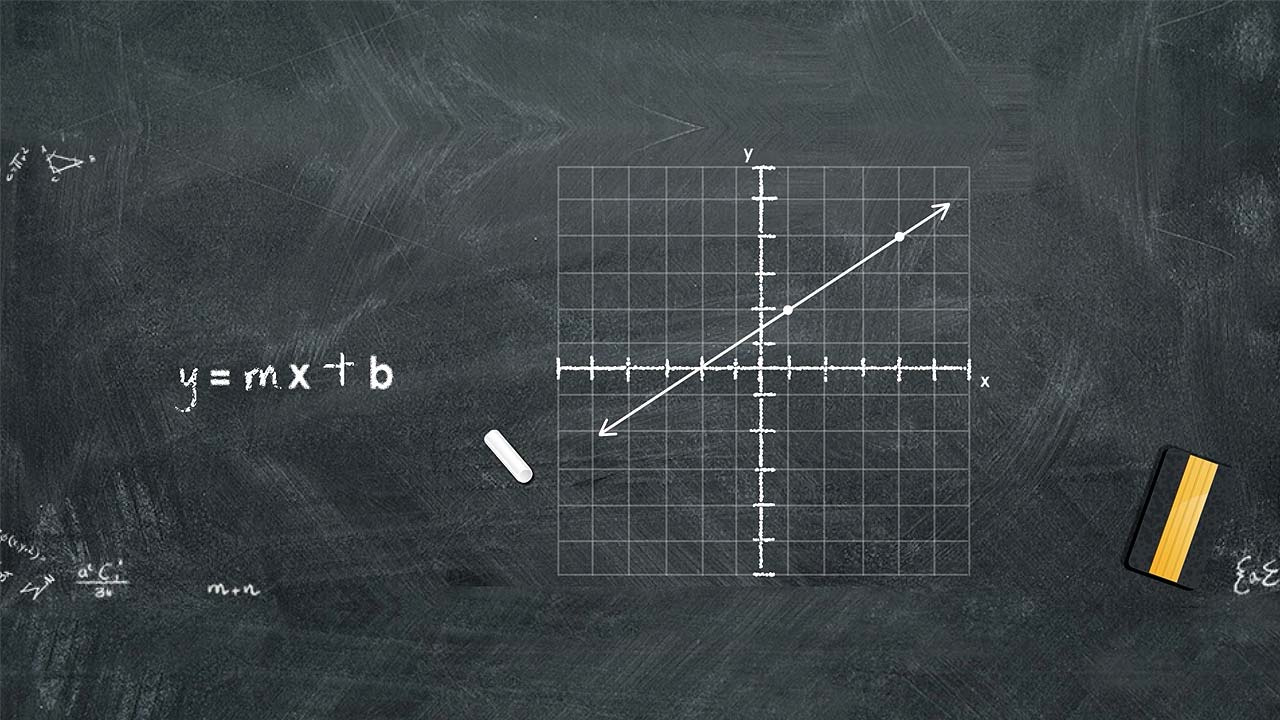# Graphing

Social/Primary ImageOverview
In science, we use equations as a tool for showing a relationship between two or more entities. Using equations allows us to create a graphic of those relationships. This graphic is known as a graph and the importance of graphing cannot be overstated. Graphs are used as a tool to observe correlations between various experimental parameters.
Objectives

Upon completion of this exercise, you will be able to:

1. Recognize the purpose of graphing lines
2. Describe how to find the slope of a line
3. Explain the equation of a line
4. Perform basic calculations to find the slope and intercepts